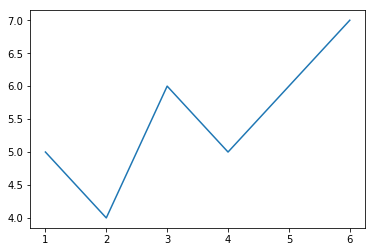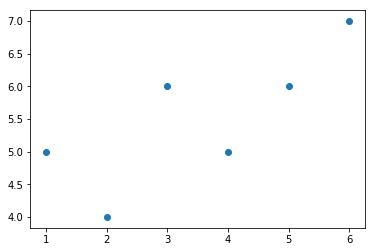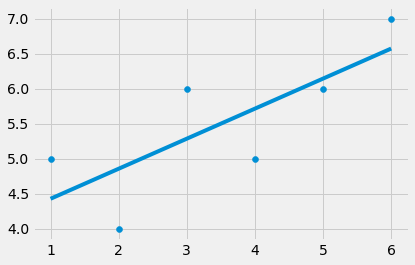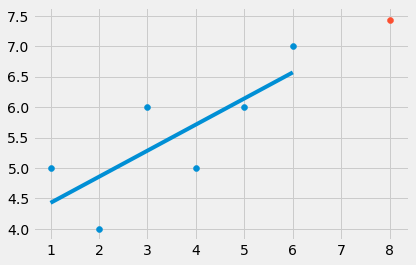January 28, 2020

Remember Me

### Create an account

Fields marked with an asterisk (*) are required.
Name *
Email *
Verify email *## linear Regression For Machine learning (with Tutorial)

ML Supervised

User Rating: 0 / 5• Definition:

Regression is for determining relationships between two or more variables & also used for Forcast new observation.

Exemple : X and Y are the variables.

when X = 5.(1,1), (2,2), (4,4), (100,100), (20, 20) , Y = 5 - the relationship between X and Y is Y = X

when X = 5.(1,1), (2,4), (4,16), (100,10000), (20, 400) , Y = 25 - the relationship ibetween X and Y is Y = X * X

In these two examples, we can determine the relationship between two given variables (X and Y) because we could easily identify the relationship between them. Overall, machine learning works in the same way.

the computer looks at some examples and then tries to identify “the most suitable” relationship between the sets X and Y. Using this identified relationship, it will try to predict for new examples the Y.

• X is termed as the independent variable: this is the variable that explains the other one.
• Y is termed as the dependent variable: this is the variable whose values we want to explain or forecast,and its a values depend on somthing else.

Q: to know Y we must use the equation : Y = mX + b what's m & b ?

A: m is the slope.

Equation of m , the best fit line: m = ((mean X.mean Y)- (mean XY)) / ((mean X)^2 - (mean X^2))

A: b is the Y_intercept. b = (mean Y) - m(mean X).

• linear regression using python:

```from statistics import mean #import the "mean" from statistics to calculate the m & b.
import numpy as np
import matplotlib.pyplot as plt #import Matplotlib to visualize Data.

xs = [1,2,3,4,5,6]
ys = [5,4,6,5,6,7]

plt.plot(xs,ys)
plt.show() #visualize the Data as Plot designe.
``````plt.scatter(xs,ys)
plt.show() #visualize the Data as scatter designe.``````xs = np.array([1,2,3,4,5,6], dtype=np.float64) #convert to numpy array and change the data type.
ys = np.array([5,4,6,5,6,7], dtype=np.float64)

def best_fit_slope(xs,ys): #define a function to calculate the m ( the slope of the best fit line
m = (((mean(xs) * mean(ys))- mean(xs*ys)) /
((mean(xs) *mean(xs)) - mean(xs*xs)))

return m

m = best_fit_slope(xs,ys)

print (m)
```

```0.42857142857142866
```

```def best_fit_slope(xs,ys):
m = (((mean(xs) * mean(ys))- mean(xs*ys)) /
((mean(xs) *mean(xs)) - mean(xs*xs)))
b = mean(ys) - m*mean(xs)     #calculate the intercept.
return m, b

m,b = best_fit_slope(xs,ys)

print (m,b)
```

```0.42857142857142866 4.0
```

```from matplotlib import style

style.use('fivethirtyeight')
regression_line = [(m*x)+b for x in xs] #create the line that fits the data X & Y.
plt.scatter(xs,ys)
plt.plot(xs, regression_line)
plt.show()
``````from matplotlib import style

style.use('fivethirtyeight')
regression_line = [(m*x)+b for x in xs]

predict_x = 8
predict_y = (m*predict_x)+b #Predict the value of Y.

plt.scatter(xs,ys)
plt.scatter(predict_x,predict_y)
plt.plot(xs, regression_line)
plt.show()
```## Scikit-learn: Machine Learning in Python

ML Supervised

User Rating: 5 / 5The Python programming language is establishing itself as one of the most popular languages for scientific computing. Thanks to its high-level interactive nature and its maturing ecosystem of scientific libraries...

## An Introduction to MCMC for Machine Learning

ML Supervised

User Rating: 0 / 5A recent survey places the Metropolis algorithm among the ten algorithms that have had the greatest influence on the development and practice of science and engineering in the 20th century (Beichl & Sullivan, 2000).

## Supervised ML: A Review of Classification Techniques

ML Supervised

User Rating: 0 / 5There are several applications for Machine Learning (ML), the most significant of which is data mining. People are often prone to making mistakes during analyses or, possibly, when trying to establish relationships between multiple features

## Digital Barrels: The Rise Of Machine Learning In Oil And Gas

ML Supervised

User Rating: 0 / 5How automisation, machine learning and artificial intelligence could save the oil industry from itself

### Popular Articles#### Pourquoi DataScienceToDay.net ?

Oct 05, 2018 Catégorie (fr-fr)

### Trending Now#### Scikit-learn: Machine Learning in Python

Apr 06, 2018 Rate: 0.00#### An Introduction to MCMC for Machine Lear…

Apr 06, 2018 Rate: 0.00#### Treillis de concepts et classification s…

Apr 08, 2018 Rate: 0.00